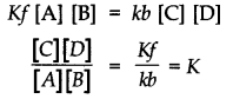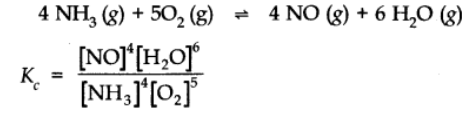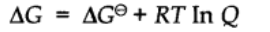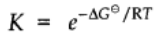At a constant temperature, the rate of a chemical reaction is directly proportional to the product of the molar concentrations of the reactants each raised to a power equal to the corresponding stoichiometric coefficients as represented by the balanced chemical equation. Let us consider the reaction,

aA + bB +… ⟶ products

Rate of reaction α [A]a[B]b……

By the law, rate of reaction = k[A]a[B]b

Here a and b are stoichiometric coefficients. K is the rate constant.

Let us consider a general reversible reaction

A + B ⇌ C + D

Applying Law of Mass Action,

Rate of the forward reaction α[A][B] = Kf[A][B]

When Kf is a constant of proportionality and is called velocity constant for the forward reaction.

Rate of the backward reaction α[C][D] = Kb[C][D]

At equilibrium,

Rate of the forward reaction = Rate of the backward reactionAt constant temperatures K is also constant and is called Equilibrium constant.

Now let us consider a more general reversible reaction in a state of equilibrium. By applying Law of mass action.## Equilibrium Constant for the reaction## Relationship between Equilibrium constant K, reaction Quotient Q and Gibbs energy G.

A mathematical expression of thermodynamic view of equilibrium can be described by time equation.where G is standard Gibbs energy.

At equilibrium when ∆G = 0Taking antilog on both sides